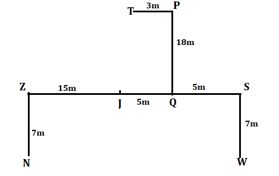Latest Banking jobs   »

# Reasoning Ability Quiz For IBPS PO Prelims 2022- 18th September

Directions (1-5): In this question, relationship between different elements is shown in the statements. The statements are followed by conclusions. Study the conclusions based on the given statement and select the appropriate answer.

Q1. Statements: A<B<C=G, G≥K<L, K≥F
Conclusions: I. G>F
II. G=F
(a) Only conclusion I is true
(b) Only conclusion II is true
(c) Either conclusion I or II is true
(d) Both conclusions I and II are true
(e) Neither conclusion I nor II is true

Q2. Statements: A = P <W < V >K > Q; B < A; K = M
Conclusions: I. M>Q
II. A > V
(a) Only conclusion I is true
(b) Only conclusion II is true
(c) Either conclusion I or II is true
(d) Both conclusions I and II are true
(e) Neither conclusion I nor II is true

Q3. Statements: A = P <W < V >K > Q; B < A; K = M
Conclusions: I. B<V
II. V>Q
(a) Only conclusion I is true
(b) Only conclusion II is true
(c) Either conclusion I or II is true
(d) Both conclusions I and II are true
(e) Neither conclusion I nor II is true

Q4. Statements: H ≤ G = B < E; B ≤ W
Conclusions: I. H < E
II. H≤B
(a) Only conclusion I is true
(b) Only conclusion II is true
(c) Either conclusion I or II is true
(d) Both conclusions I and II are true
(e) Neither conclusion I nor II is true

Q5. Statements: M <U ≤ Q < L = V < W ≥ Y; B = V ≤ R
Conclusions: I. Y< B
II. R < Q
(a) Only conclusion I is true
(b) Only conclusion II is true
(c) Either conclusion I or II is true
(d) Both conclusions I and II are true
(e) Neither conclusion I nor II is true

Direction (6-10): Study the given information and answer the following question.
Point P is 18m north of point Q. Point S is 5m east of point Q. Point Z is west of 15m of point J. Point J is 5m west of point Q. Point N is 7m south of point Z. Point S is 7m north of point W. Point P is 3m east of point T

Q6. What is the shortest distance between N and W?
(a) 20m
(b)15m
(c) 10m
(d)5m
(e)25m

Q7. Point Z is in which direction with respect to S?
(a) North-East
(b) North-west
(c) East
(d) South
(e) None of these

Q8. What is the direction of Point T with respect of point S?
(a) East
(b) South
(c) West
(d) North-west
(e) None of these

Q9. In which direction of Point J with respect to Point T?
(a) North-east
(b) North
(c) West
(d) South-west
(e) None of these

Q10. If Point K is 5m west of point P then what is the shortest distance between Point K and Point J?
(a) 10m
(b) 9m
(c) 11m
(d) 6m
(e) None of these

Q11. If all the letters in the word PAINTBALL are arranged in alphabetical order from left to right in such a way that vowels are arranged first followed by consonants, then how many letters are there in between I and N after the arrangement?
(a) Two
(b) Three
(c) None
(d) One
(e) Four

Q12. If in the number 82164739, 1 is added to each of the digit which is less than five and 1 is subtracted from each of the digit which is greater than five then how many digits are repeating in the number thus formed?
(a) One
(b) Two
(c) None
(d) Three
(e) Four

Q13. The position of how many alphabets will remain unchanged if each of the alphabets in the word ‘PARANORMAL’ is arranged in alphabetical order from left to right?
(a) Two
(b) One
(c) None
(d) Three
(e) Four

Q14. Which of the following elements should come in a place ‘?’ ?
JK12 LM14 NO16 PQ18 ?
(a) ST21
(b) RS20
(c) TU22
(d) UV23
(e) None of these

Q15. Sumit is 18th from the left end of a row and Adarsh is 10th from the right end of row. If they interchanged their positions then adarsh ranks become 22 from right end. Find total number of persons in the row?
(a) 40
(b) 39
(c) 38
(d) 41
(e) None of these

Solutions

S1. Ans. (c)
Sol. I. G>F (false)
II. G=F (false)

S2. Ans. (a)
Sol. I. M>Q(true)
II. A > V(false)

S3. Ans. (d)
Sol. I. B<V(true)
II. V>Q(true)

S4. Ans. (d)
Sol. I. H<E(true)
II. H≤B(true)

S5. Ans. (e)
Sol. I. Y< B(false)
II. R < Q(false)

Solution (6-10):
Sol.S6. Ans. (e)
S7. Ans. (e)
S8. Ans. (d)
S9. Ans. (d)
S10. Ans. (e)

S11. Ans. (b)

S12. Ans. (a)

S13. Ans. (b)

S14. Ans. (b)

S15. Ans. (b)
Sol. Total number of persons in the row= (18+22-1) = 39#### Congratulations!Download Hindu Review of October 2021: Free PDF